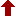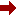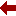#### SLIM21

 Sort Order Chronological Author Title ItemType,Author ItemType,Title Display Format Tabular Publication Bibliography Items / Page 5 10 25

Click the serial number on the left to view the details of the item.
#  AuthorTitleAccn#YearItem Type Claims
1 Yoshida, Kentaroh Yang???Baxter Deformation of 2D Non-Linear Sigma Models I11819 2021 eBook
2 Schweizer, Wolfgang Special Functions in Physics with MATLAB I11728 2021 eBook
3 Nagao, Hidehito Pad?? Methods for Painlev?? Equations I11676 2021 eBook
4 Akhmedova, Valeriya Selected Special Functions for Fundamental Physics I09479 2019 eBook
5 Pastras, Georgios The Weierstrass Elliptic Function and Applications in Classical and Quantum Mechanics I09024 2020 eBook
6 Mathai, A.M The H-Function I05634 2010 eBook
7 Araki, Huzihiro Quantum and Non-Commutative Analysis I04479 1993 eBook
8 Terziogammalu, T Advances in the Theory of Fr??chet Spaces I03867 1989 eBook
9 Avery, John S Hyperspherical Harmonics I03066 1989 eBook
10 Nevai, Paul Orthogonal Polynomials I02652 1990 eBook
 (page:1 / 2) [#19] Next Page Last Page

1.TitleYang???Baxter Deformation of 2D Non-Linear Sigma Models : Towards Applications to AdS/CFT
Author(s)Yoshida, Kentaroh
PublicationSingapore, Springer Nature Singapore, 2021.
DescriptionXII, 70 p. 2 illus : online resource
Abstract NoteIn mathematical physics, one of the fascinating issues is the study of integrable systems. In particular, non-perturbative techniques that have been developed have triggered significant insight for real physics. There are basically two notions of integrability: classical integrability and quantum integrability. In this book, the focus is on the former, classical integrability. When the system has a finite number of degrees of freedom, it has been well captured by the Arnold???Liouville theorem. However, when the number of degrees of freedom is infinite, as in classical field theories, the integrable structure is enriched profoundly. In fact, the study of classically integrable field theories has a long history and various kinds of techniques, including the classical inverse scattering method, which have been developed so far. In previously published books, these techniques have been collected and well described and are easy to find in traditional, standard textbooks. One of the intriguing subjects in classically integrable systems is the investigation of deformations preserving integrability. Usually, it is not considered systematic to perform such a deformation, and one must study systems case by case and show the integrability of the deformed systems by constructing the associated Lax pair or action-angle variables. Recently, a new, systematic method to perform integrable deformations of 2D non-linear sigma models was developed. It was invented by C. Klimcik in 2002, and the integrability of the deformed sigma models was shown in 2008. The original work was done for 2D principal chiral models, but it has been generalized in various directions nowadays. In this book, the recent progress on this Yang???Baxter deformation is described in a pedagogical manner, including some simple examples. Applications of Yang???Baxter deformation to string theory are also described briefly.
ISBN,Price9789811617034
Keyword(s)1. DIFFERENTIAL EQUATIONS 2. EBOOK 3. EBOOK - SPRINGER 4. MATHEMATICAL PHYSICS 5. SPECIAL FUNCTIONS
Item TypeeBook
Circulation Data
 Accession# Call# Status Issued To Return Due On Physical Location I11819 On Shelf

2.TitleSpecial Functions in Physics with MATLAB
Author(s)Schweizer, Wolfgang
PublicationCham, Springer International Publishing, 2021.
DescriptionXVII, 282 p. 49 illus., 17 illus. in color : online resource
Abstract NoteThis handbook focuses on special functions in physics in the real and complex domain. It covers more than 170 different functions with additional numerical hints for efficient computation, which are useful to anyone who needs to program with other programming languages as well. The book comes with MATLAB-based programs for each of these functions and a detailed html-based documentation. Some of the explained functions are: Gamma and Beta functions; Legendre functions, which are linked to quantum mechanics and electrodynamics; Bessel functions; hypergeometric functions, which play an important role in mathematical physics; orthogonal polynomials, which are largely used in computational physics; and Riemann zeta functions, which play an important role, e.g., in quantum chaos or string theory. The book???s primary audience are scientists, professionals working in research areas of industries, and advanced students in physics, applied mathematics, and engineering
ISBN,Price9783030642327
Keyword(s)1. Computational Mathematics and Numerical Analysis 2. Computational Physics and Simulations 3. COMPUTER SIMULATION 4. EBOOK 5. EBOOK - SPRINGER 6. MATHEMATICAL PHYSICS 7. Mathematics???Data processing 8. SPECIAL FUNCTIONS 9. Theoretical, Mathematical and Computational Physics
Item TypeeBook
Circulation Data
 Accession# Call# Status Issued To Return Due On Physical Location I11728 On Shelf

3.PublicationSingapore, Springer Nature Singapore, 2021.
DescriptionVIII, 90 p. 2 illus., 1 illus. in color : online resource
Abstract NoteThe isomonodromic deformation equations such as the Painlev?? and Garnier systems are an important class of nonlinear differential equations in mathematics and mathematical physics. For discrete analogs of these equations in particular, much progress has been made in recent decades. Various approaches to such isomonodromic equations are known: the Painlev?? test/Painlev?? property, reduction of integrable hierarchy, the Lax formulation, algebro-geometric methods, and others. Among them, the Pad?? method explained in this book provides a simple approach to those equations in both continuous and discrete cases. For a given function f(x), the Pad?? approximation/interpolation supplies the rational functions P(x), Q(x) as approximants such as f(x)~P(x)/Q(x). The basic idea of the Pad?? method is to consider the linear differential (or difference) equations satisfied by P(x) and f(x)Q(x). In choosing the suitable approximation problem, the linear differential equations give the Lax pair for some isomonodromic equations. Although this relation between the isomonodromic equations and Pad?? approximations has been known classically, a systematic study including discrete cases has been conducted only recently. By this simple and easy procedure, one can simultaneously obtain various results such as the nonlinear evolution equation, its Lax pair, and their special solutions. In this way, the method is a convenient means of approaching the isomonodromic deformation equations
ISBN,Price9789811629983
Keyword(s)1. ANALYSIS 2. EBOOK 3. EBOOK - SPRINGER 4. MATHEMATICAL ANALYSIS 5. MATHEMATICAL PHYSICS 6. SPECIAL FUNCTIONS
Item TypeeBook
Circulation Data
 Accession# Call# Status Issued To Return Due On Physical Location I11676 On Shelf

4.TitleSelected Special Functions for Fundamental Physics
Author(s)Akhmedova, Valeriya;Akhmedov, Emil T
PublicationCham, Springer International Publishing, 2019.
DescriptionXI, 116 p. 20 illus : online resource
Abstract NoteThis book presents calculation methods that are used in both mathematical and theoretical physics. These methods will allow readers to work with selected special functions and more generally with differential equations, which are the most frequently used in quantum mechanics, theory of relativity and quantum field theory. The authors explain various approximation methods used to solve differential equations and to estimate integrals. They also address the basics of the relations between differential equations, special functions and representation theory of some of the simplest algebras on the one hand, and fundamental physics on the other. Based on a seminar for graduate physics students, the book offers a compact and quick way to learn about special functions. To gain the most from it, readers should be familiar with the basics of calculus, linear algebra, and complex analysis, as well as the basic methods used to solve differential equations and calculate integrals.
ISBN,Price9783030350895
Keyword(s)1. EBOOK 2. EBOOK - SPRINGER 3. Mathematical Applications in the Physical Sciences 4. Mathematical Methods in Physics 5. MATHEMATICAL PHYSICS 6. PHYSICS 7. SPECIAL FUNCTIONS
Item TypeeBook
Circulation Data
 Accession# Call# Status Issued To Return Due On Physical Location I09479 On Shelf

5.TitleThe Weierstrass Elliptic Function and Applications in Classical and Quantum Mechanics : A Primer for Advanced Undergraduates
Author(s)Pastras, Georgios
PublicationCham, Springer International Publishing, 2020.
DescriptionXIII, 111 p. 14 illus., 13 illus. in color : online resource
Abstract NoteThe field of elliptic functions, apart from its own mathematical beauty, has many applications in physics in a variety of topics, such as string theory or integrable systems. This book, which focuses on the Weierstrass theory of elliptic functions, aims at senior undergraduate and junior graduate students in physics or applied mathematics. Supplemented by problems and solutions, it provides a fast, but thorough introduction to the mathematical theory and presents some important applications in classical and quantum mechanics. Elementary applications, such as the simple pendulum, help the readers develop physical intuition on the behavior of the Weierstrass elliptic and related functions, whereas more Interesting and advanced examples, like the n=1 Lam?? problem-a periodic potential with an exactly solvable band structure, are also presented
ISBN,Price9783030593858
Keyword(s)1. Applications of Mathematics 2. APPLIED MATHEMATICS 3. CLASSICAL MECHANICS 4. EBOOK 5. EBOOK - SPRINGER 6. ENGINEERING MATHEMATICS 7. MATHEMATICAL PHYSICS 8. MECHANICS 9. NUCLEAR PHYSICS 10. Particle and Nuclear Physics 11. SPECIAL FUNCTIONS 12. Theoretical, Mathematical and Computational Physics
Item TypeeBook
Circulation Data
 Accession# Call# Status Issued To Return Due On Physical Location I09024 On Shelf

6.TitleThe H-Function : Theory and Applications
Author(s)Mathai, A.M;Saxena, Ram Kishore;Haubold, Hans J
PublicationNew York, NY, Springer New York, 2010.
DescriptionXIV, 268 p : online resource
Abstract NoteThe topics of special H-function and fractional calculus are currently undergoing rapid changes both in theory and application. Taking into account the latest research results, the authors delve into these topics as they relate to applications to problems in statistics, physics, and engineering, particularly in condensed matter physics, plasma physics, and astrophysics. The book sets forth the definitions, contours, existence conditions, and particular cases for the H-function, then explores the properties and relationships among the Laplace, Fourier, Hankel, and other transforms. From here, the H-functions are utilized for applications in statistical distribution theory, structures of random variables, generalized distributions, Mathai???s pathway models, and versatile integrals. Functions of matrix argument are introduced with a focus on real-valued scalar functions when the matrices are real or Hermitian positive-definite. The text concludes with important recent applications to physical problems in reaction, diffusion, reaction-diffusion theory and statistics, and superstatistics. Generalized entropies as well as applications in astrophysics are dealt with. Over the last few years, material in this book has been added to various courses and developed to meet the needs of scholars at the PhD level. All exercises in the book have been used to probe the knowledge and ability of mathematics, statistics, and physics to students and researchers.
ISBN,Price9781441909169
Keyword(s)1. APPLIED MATHEMATICS 2. EBOOK 3. EBOOK - SPRINGER 4. ENGINEERING MATHEMATICS 5. FUNCTIONAL ANALYSIS 6. Mathematical and Computational Engineering 7. Mathematical Methods in Physics 8. MATHEMATICAL PHYSICS 9. PHYSICS 10. SPECIAL FUNCTIONS 11. Statistical Theory and Methods 12. Statistics?? 13. Theoretical, Mathematical and Computational Physics
Item TypeeBook
Circulation Data
 Accession# Call# Status Issued To Return Due On Physical Location I05634 On Shelf

7.TitleQuantum and Non-Commutative Analysis : Past, Present and Future Perspectives
Author(s)Araki, Huzihiro;Ito, Keiichi R;Kishimoto, Akitaka;Ojima, Izumi
PublicationDordrecht, Springer Netherlands, 1993.
DescriptionXII, 464 p : online resource
Abstract NoteIn the past decade, there has been a sudden and vigorous development in a number of research areas in mathematics and mathematical physics, such as theory of operator algebras, knot theory, theory of manifolds, infinite dimensional Lie algebras and quantum groups (as a new topics), etc. on the side of mathematics, quantum field theory and statistical mechanics on the side of mathematical physics. The new development is characterized by very strong relations and interactions between different research areas which were hitherto considered as remotely related. Focussing on these new developments in mathematical physics and theory of operator algebras, the International Oji Seminar on Quantum Analysis was held at the Kansai Seminar House, Kyoto, JAPAN during June 25-29, 1992 by a generous sponsorship of the Japan Society for the Promotion of Science and the Fujihara Foundation of Science, as a workshop of relatively small number of (about 50) invited participants. This was followed by an open Symposium at RIMS, described below by its organizer, A. Kishimoto. The Oji Seminar began with two key-note addresses, one by V.F.R. Jones on Spin Models in Knot Theory and von Neumann Algebras and by A. Jaffe on Where Quantum Field Theory Has Led. Subsequently topics such as Subfactors and Sector Theory, Solvable Models of Statistical Mechanics, Quantum Field Theory, Quantum Groups, and Renormalization Group Ap?? proach, are discussed. Towards the end, a panel discussion on Where Should Quantum Analysis Go? was held
ISBN,Price9789401728232
Keyword(s)1. Associative rings 2. Associative Rings and Algebras 3. EBOOK 4. EBOOK - SPRINGER 5. Elementary particles (Physics) 6. Elementary Particles, Quantum Field Theory 7. GROUP THEORY 8. Group Theory and Generalizations 9. QUANTUM FIELD THEORY 10. Rings (Algebra) 11. SPECIAL FUNCTIONS
Item TypeeBook
Circulation Data
 Accession# Call# Status Issued To Return Due On Physical Location I04479 On Shelf

8.TitleAdvances in the Theory of Fr??chet Spaces
Author(s)Terziogammalu, T
PublicationDordrecht, Springer Netherlands, 1989.
DescriptionXVIII, 364 p : online resource
Abstract NoteFrechet spaces have been studied since the days of Banach. These spaces, their inductive limits and their duals played a prominent role in the development of the theory of locally convex spaces. Also they are natural tools in many areas of real and complex analysis. The pioneering work of Grothendieck in the fifties has been one of the important sources of inspiration for research in the theory of Frechet spaces. A structure theory of nuclear Frechet spaces emerged and some important questions posed by Grothendieck were settled in the seventies. In particular, subspaces and quotient spaces of stable nuclear power series spaces were completely characterized. In the last years it has become increasingly clear that the methods used in the structure theory of nuclear Frechet spaces actually provide new insight to linear problems in diverse branches of analysis and lead to solutions of some classical problems. The unifying theme at our Workshop was the recent developments in the theory of the projective limit functor. This is appropriate because of the important role this theory had in the recent research. The main results of the structure theory of nuclear Frechet spaces can be formulated and proved within the framework of this theory. A major area of application of the theory of the projective limit functor is to decide when a linear operator is surjective and, if it is, to determine whether it has a continuous right inverse
ISBN,Price9789400924567
Keyword(s)1. EBOOK 2. EBOOK - SPRINGER 3. FUNCTIONS OF COMPLEX VARIABLES 4. OPERATOR THEORY 5. PARTIAL DIFFERENTIAL EQUATIONS 6. Several Complex Variables and Analytic Spaces 7. SPECIAL FUNCTIONS
Item TypeeBook
Circulation Data
 Accession# Call# Status Issued To Return Due On Physical Location I03867 On Shelf

9.TitleHyperspherical Harmonics : Applications in Quantum Theory
Author(s)Avery, John S
PublicationDordrecht, Springer Netherlands, 1989.
DescriptionXVI, 256 p : online resource
Abstract Notewhere d 3 3)2 ( L x - -- i3x j3x j i i>j Thus the Gegenbauer polynomials play a role in the theory of hyper spherical harmonics which is analogous to the role played by Legendre polynomials in the familiar theory of 3-dimensional spherical harmonics; and when d = 3, the Gegenbauer polynomials reduce to Legendre polynomials. The familiar sum rule, in 'lrlhich a sum of spherical harmonics is expressed as a Legendre polynomial, also has a d-dimensional generalization, in which a sum of hyper spherical harmonics is expressed as a Gegenbauer polynomial (equation (3-27??: The hyper spherical harmonics which appear in this sum rule are eigenfunctions of the generalized angular monentum 2 operator A , chosen in such a way as to fulfil the orthonormality relation: VIe are all familiar with the fact that a plane wave can be expanded in terms of spherical Bessel functions and either Legendre polynomials or spherical harmonics in a 3-dimensional space. Similarly, one finds that a d-dimensional plane wave can be expanded in terms of HYPERSPHERICAL HARMONICS xii "hyperspherical Bessel functions" and either Gegenbauer polynomials or else hyperspherical harmonics (equations ( 4 - 27) and ( 4 - 30) ) : 00 ik??x e = (d-4)!!A~oiA(d+2A-2)j~(kr)C~(~k'~) 00 (d-2)!!I(0) 2: iAj~(kr) 2:Y~ (["2k)Y (["2) A A=O ). l). l)J where I(O) is the total solid angle. This expansion of a d-dimensional plane wave is useful when we wish to calculate Fourier transforms in a d-dimensional space
ISBN,Price9789400923232
Keyword(s)1. Atomic, Molecular, Optical and Plasma Physics 2. ATOMS 3. EBOOK 4. EBOOK - SPRINGER 5. PHYSICAL CHEMISTRY 6. PHYSICS 7. QUANTUM PHYSICS 8. SPECIAL FUNCTIONS
Item TypeeBook
Circulation Data
 Accession# Call# Status Issued To Return Due On Physical Location I03066 On Shelf

10.TitleOrthogonal Polynomials : Theory and Practice
Author(s)Nevai, Paul
PublicationDordrecht, Springer Netherlands, 1990.
Description488 p : online resource
Abstract NoteThis volume contains the Proceedings of the NATO Advanced Study Institute on "Orthogonal Polynomials and Their Applications" held at The Ohio State University in Columbus, Ohio, U.S.A. between May 22,1989 and June 3,1989. The Advanced Study Institute primarily concentrated on those aspects of the theory and practice of orthogonal polynomials which surfaced in the past decade when the theory of orthogonal polynomials started to experience an unparalleled growth. This progress started with Richard Askey's Regional Confer?? ence Lectures on "Orthogonal Polynomials and Special Functions" in 1975, and subsequent discoveries led to a substantial revaluation of one's perceptions as to the nature of orthogonal polynomials and their applicability. The recent popularity of orthogonal polynomials is only partially due to Louis de Branges's solution of the Bieberbach conjecture which uses an inequality of Askey and Gasper on Jacobi polynomials. The main reason lies in their wide applicability in areas such as Pade approximations, continued fractions, Tauberian theorems, numerical analysis, probability theory, mathematical statistics, scattering theory, nuclear physics, solid state physics, digital signal processing, electrical engineering, theoretical chemistry and so forth. This was emphasized and convincingly demonstrated during the presentations by both the principal speakers and the invited special lecturers. The main subjects of our Advanced Study Institute included complex orthogonal polynomials, signal processing, the recursion method, combinatorial interpretations of orthogonal polynomials, computational problems, potential theory, Pade approximations, Julia sets, special functions, quantum groups, weighted approximations, orthogonal polynomials associated with root systems, matrix orthogonal polynomials, operator theory and group representations
ISBN,Price9789400905016
Keyword(s)1. Computational Mathematics and Numerical Analysis 2. Computer mathematics 3. EBOOK 4. EBOOK - SPRINGER 5. FOURIER ANALYSIS 6. SPECIAL FUNCTIONS
Item TypeeBook